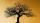# Boys and girls

There are 28 girls in the hall. 5/7 of all children are boys. How many children and how many boys are there?

Result

c =  70
s =  98

#### Solution:

c+28 = s
5/7 s = c

c-s = -28
7c-5s = 0

c = 70
s = 98

Calculated by our linear equations calculator.

Leave us a comment of example and its solution (i.e. if it is still somewhat unclear...):

Showing 0 comments:Be the first to comment!#### To solve this verbal math problem are needed these knowledge from mathematics:

Need help calculate sum, simplify or multiply fractions? Try our fraction calculator. Do you have a linear equation or system of equations and looking for its solution? Or do you have quadratic equation?

## Next similar examples:

1. The bunsKate, Zofia and Peter Liked buns. Even today, their grandmother prepare their favorite meal. Katka eats 4 bunches, Žofia 3 and Petra eats 5 buns. Their grandmother said to them, "My inmate will you know how many buns I have been make today, if those you ea
2. PearsThere were pears in the basket, I took two-fifths of them, and left six in the basket. How many pears did I take?
3. TreesFrom the total number of trees in the orchard, there are two-fifths pearls and apples are three eighty. The rest of the trees are 9 ceremonial. How many trees are in the set?
4. Orchard3/5 of the trees in the orchard are apples, 1/3 are cherries and the remaining five trees are pear. How many trees are in the orchard?
5. ClassroomOne-eighth of 9th class was interested in studying at a grammar school, at a business academy one sixth, at secondary vocational schools quarter, to SOU one third and the remaining three students were interested in the school of art direction. How many st
6. Cleaning windowsCleaning company has to wash all the windows of the school. The first day washes one-sixth of the windows of the school, the next day three more windows than the first day and the remaining 18 windows washes on the third day. Calculate how many windows ha
7. FruitsAmy bought a basket of fruits 1/5 of them were apples,1/4 were oranges, and the rest were 33 bananas. How many fruits did she buy in all?
8. EqnSolve equation with fractions: 2x/3-50=40+x/4
9. Unknown numberI think the number - its sixth is 3 smaller than its third.
10. Simple equation 324 = n • 27, solve for n
11. EquationFind x: x + 1/2 = 1/3
12. Equation with xSolve the following equation: 2x- (8x + 1) - (x + 2) / 5 = 9
13. FractionsThree-quarters of an unknown number are 4/5. What is 5/6 of this unknown number?
14. Find unknownFind unknown numerator: 4/8 + _/8 = 1
15. Unknown number 6Determine x if 1/6 of x is equal to 2/5 of the number 24.
16. GivenGiven 2x =0.125 find the value of x
17. Simple equationSolve for x: 3(x + 2) = x - 18